Note: 本文基于 3GPP Rel15 (2020-09) 版本规范。

SRS 用于上行信道信息获取、满足信道互易性时的下行信道信息获取以及上行波束管理。

NR 定义了 3 种类型的 SRS 传输：周期性 SRS，半持续性 SRS 和非周期性 SRS，通过为 SRS 资源集和 SRS 资源配置关于时域类型的高层参数 resourceType 来实现。

SRS 资源集配置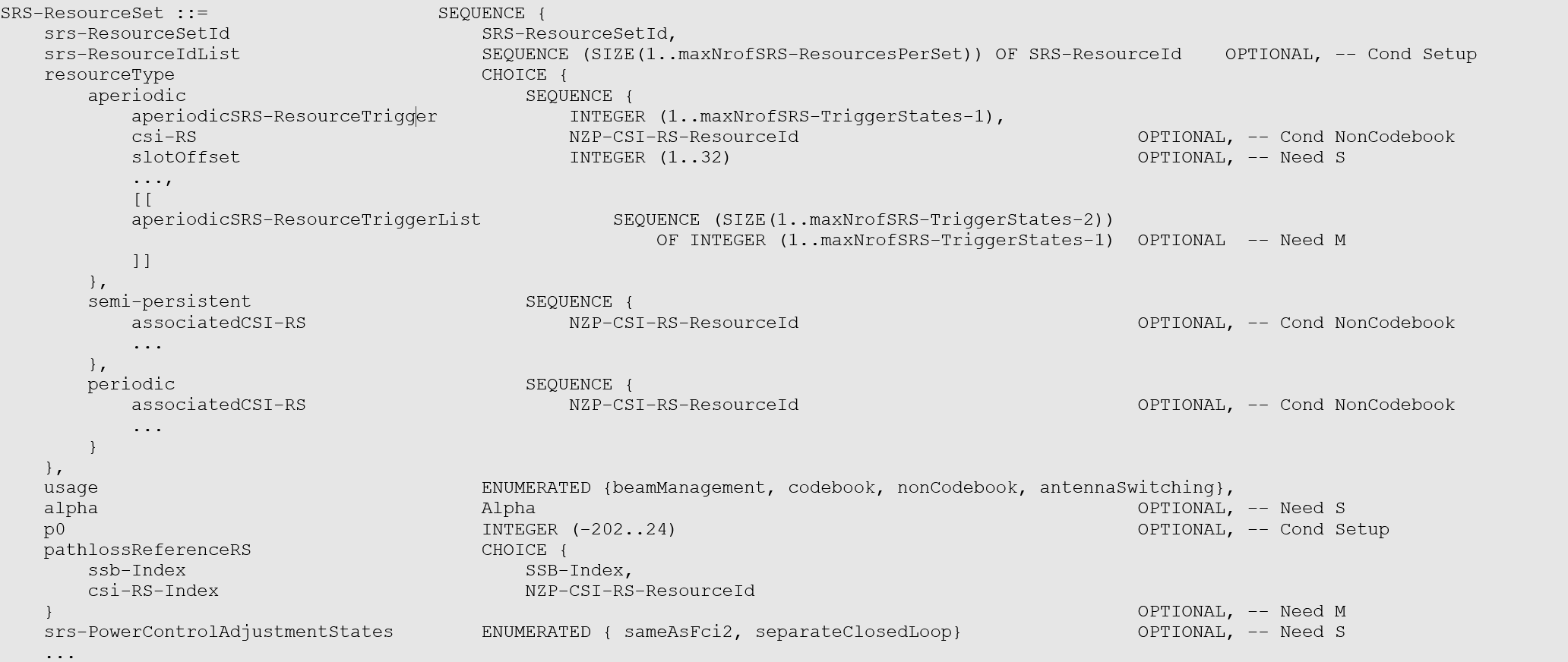SRS 资源配置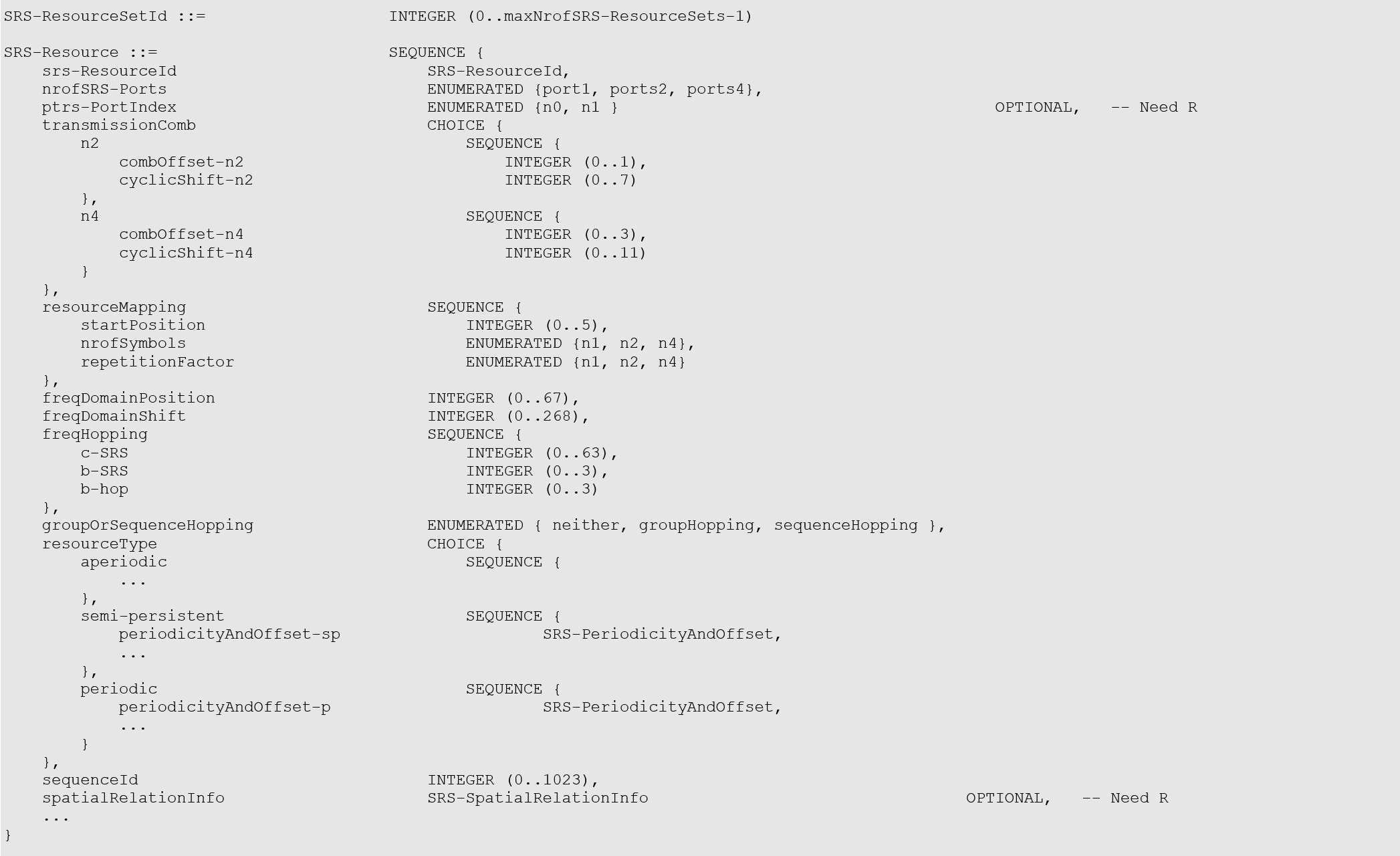• 周期性 SRS。时域类型被配置为周期 SRS 资源的所有参数由高层信令配置，UE根据所配置的参数进行周期性发送。同一个 SRS 资源集内的所有 SRS 资源具有相同的周期性。考虑到 NR 系统支持各种子载波间隔，不同子载波间隔对应的时隙时长不同，周期 SRS 资源的周期以及周期内的偏移以时隙为单位进行配置。周期 SRS 资源可配置的最小周期为 1 个时隙，最大周期为 2560 个时隙。
• 半持续性 SRS。时域类型被配置为半持续 SRS 资源在激活期间也是周期性发送。它与周期性 SRS 的区别在于 UE 在接收到关于半持续 SRS 资源的高层信令配置后不发送 SRS，只有在接收到 MAC 层发送的关于半持续 SRS 资源的激活信令后才开始周期性地发送半持续 SRS 资源对应的 SRS ，在收到 MAC 层发送的半持续 SRS 资源的去激活命令后停止发送 SRS。因此，相对于周期性 SRS 资源，半持续 SRS 资源的配置以及激活、去激活相比高层信令（RRC信令）更快，更灵活，适用于要求时延较低的业务的快速传输。与周期性 SRS 资源类似，基站通过高层信令为半持续 SRS 资源配置周期和周期内的偏移，同一个 SRS 资源集内的所有SRS资源具有相同的周期性。
• 非周期性 SRS。时域类型被配置为非周期 SRS 资源通过 DCI 信令激活。UE 每接收到一次触发非周期 SRS 资源的 SRS 触发信令，UE 进行一次所触发的 SRS 资源对应的 SRS 发送。DCI 中的 SRS 触发信令包含 2 个比特（如表 Table 7.3.1.1.2-24 所示），2 个比特可表示的 4 个状态。其中中的 1 个状态表示不触发非周期 SRS 发送，其他 3 个状态分别表示触发第一、第二、第三个 SRS 资源组；一个状态可以触发一个或多个 SRS 资源集，一个状态对应的多个 SRS 资源集可以对应多个载波。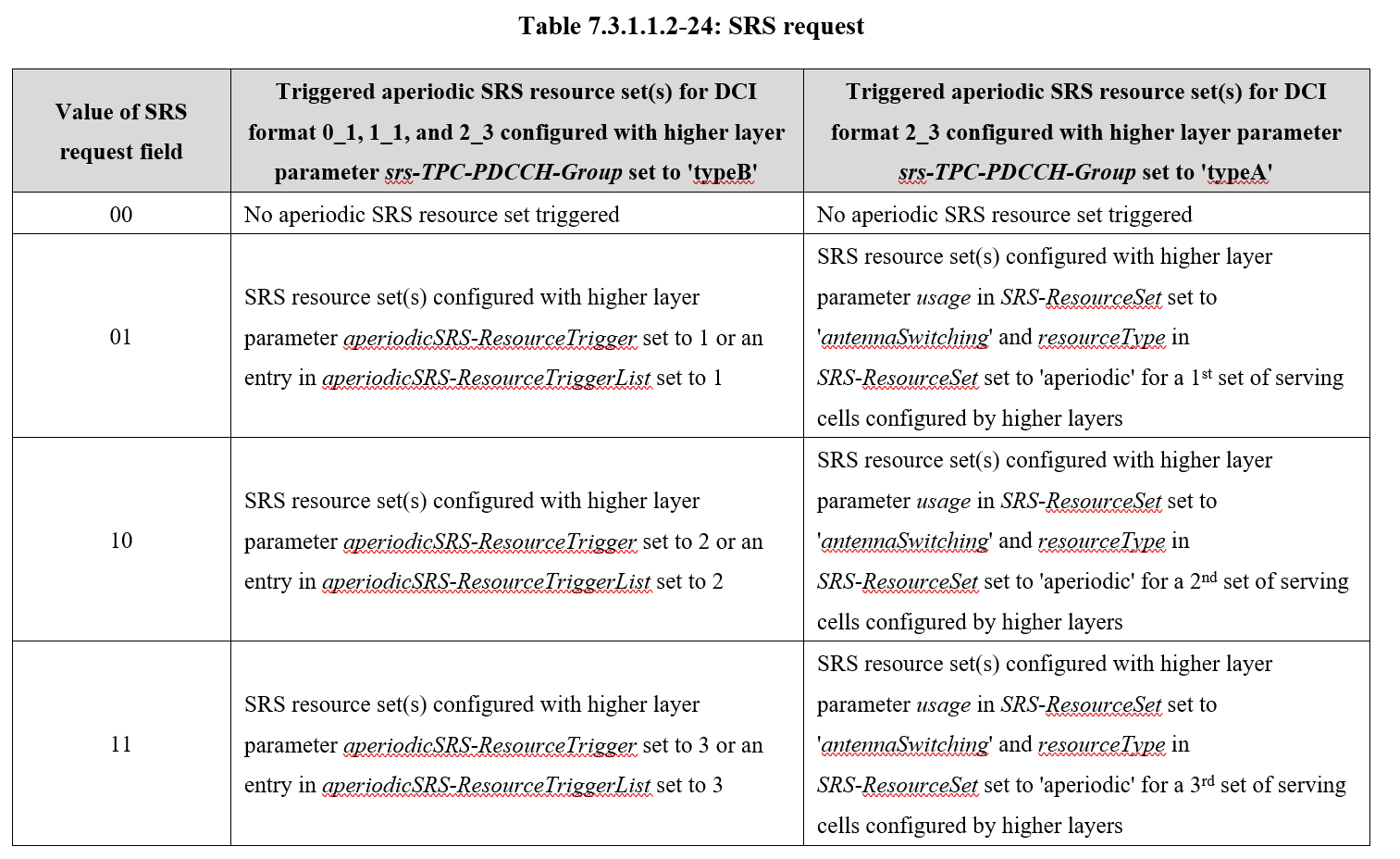# SRS 资源定义

SRS 资源由「天线端口数目 $$N_{\mathrm{ap}}^{\mathrm{SRS}}$$，连续的 OFDM 符号数目 $$N_{symb}^{SRS}$$，时域起始符号 $$\ l_0$$ 和频域起始位置 $$k_0$$」四个信息共同确定。

• 如果高层参数 SRS-ResourceSet 中的 usage 未配置为 “nonCodebook”，则 $$N_{\mathrm{ap}}^{\mathrm{SRS}}$$ 由 nrofSRS-Ports 确定。

• 如果高层参数 SRS-ResourceSet 中的 usage 配置为 “nonCodebook”，则 $$N_{\mathrm{ap}}^{\mathrm{SRS}}$$ 取决于 section 5.2.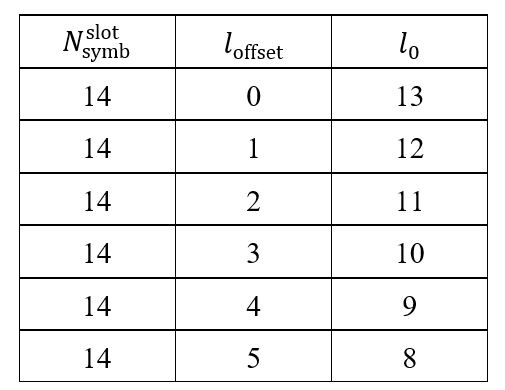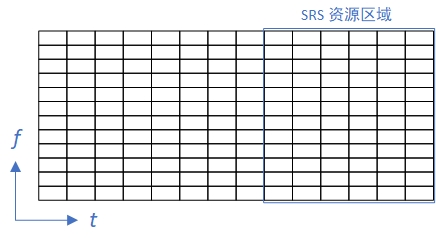# SRS 序列生成

SRS 序列基于Zadoff-Chu 序列产生：

$r^{\left(p_i\right)}\left(n,l'\right)=r_{u,v}^{\left(\alpha_i,\delta\right)}\left(n\right)$

$0\le n\le M_{\mathrm{sc},b}^{\mathrm{SRS}}-1$

$l'\in\left\{0,1,\ldots,N_{\mathrm{symb}}^{\mathrm{SRS}}-1\right\}$

$$r_{u,v}^{\left(\alpha_i,\delta\right)}\left(n\right)$$ 是 扩展 ZC 序列，序列长度为 $$M_{\mathrm{sc},b}^{\mathrm{SRS}}$$，其中 $$\delta={\mathrm{log}}_\mathrm{2}\left(K_{\mathrm{TC}}\right)$$$$\alpha_i$$ 是对应于天线端口 $$p_i$$ 的循环移位。$$l'$$ 是 SRS 资源的 OFDM 符号索引。传输梳齿数目 $$K_{\mathrm{TC}}$$ 由高层参数 transmissionComb 确定，循环移位 $$\alpha_i$$ 由下式给出：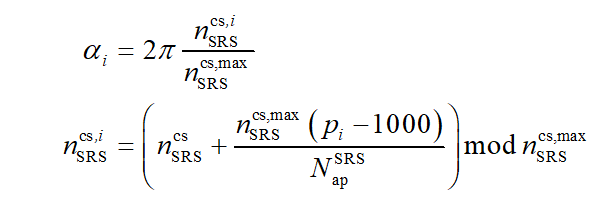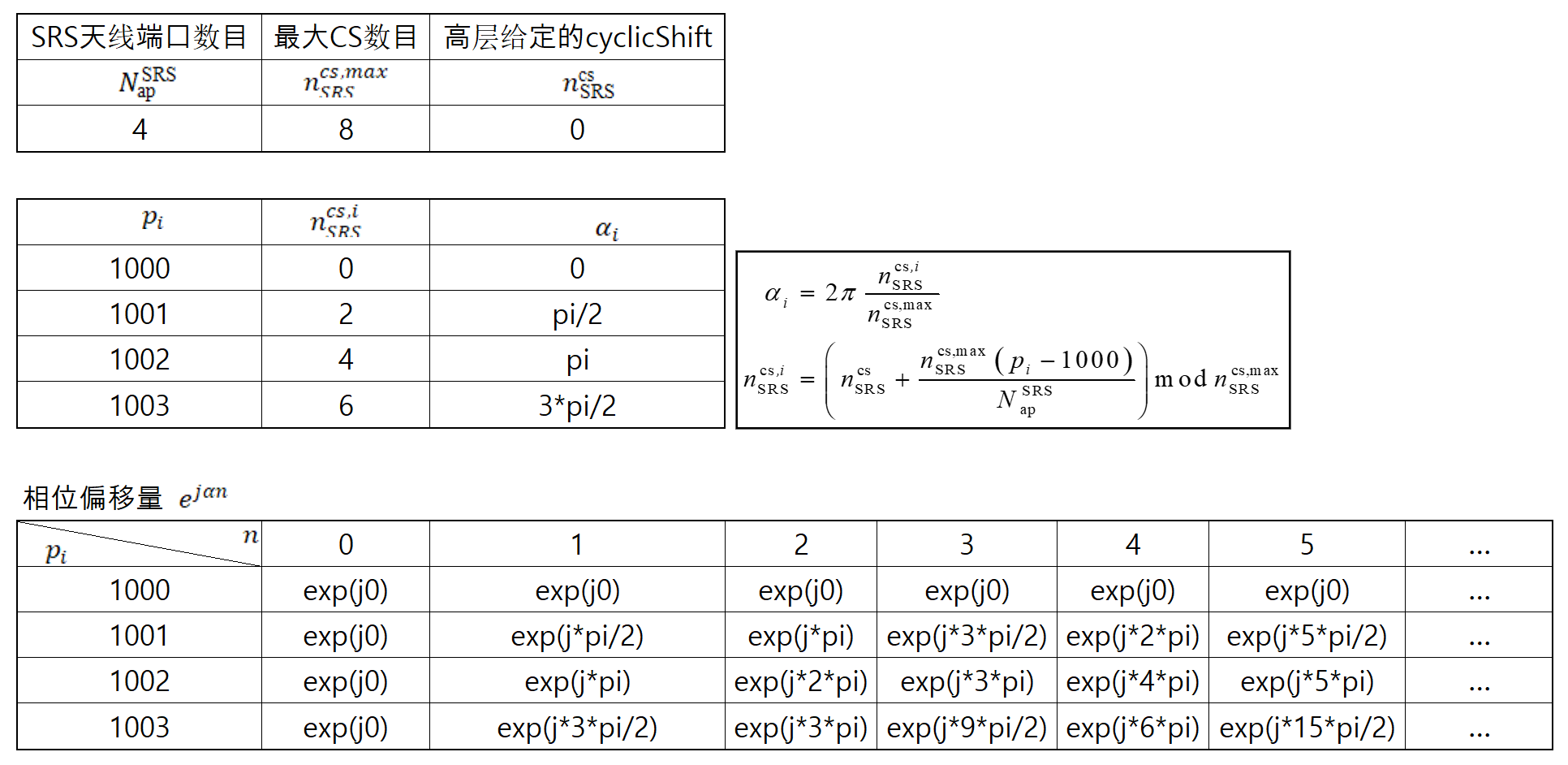SRS 的基序列被分成若干组，每组包含若干序列。如果基站给终端配置为 groupOrSequenceHopping = neither，则每次终端发送的 SRS 序列不变；如果基站给终端配置为 groupOrSequenceHopping = groupHopping or sequenceHopping，则每次终端发送上行 SRS 时按照以下规则采用不同的序列。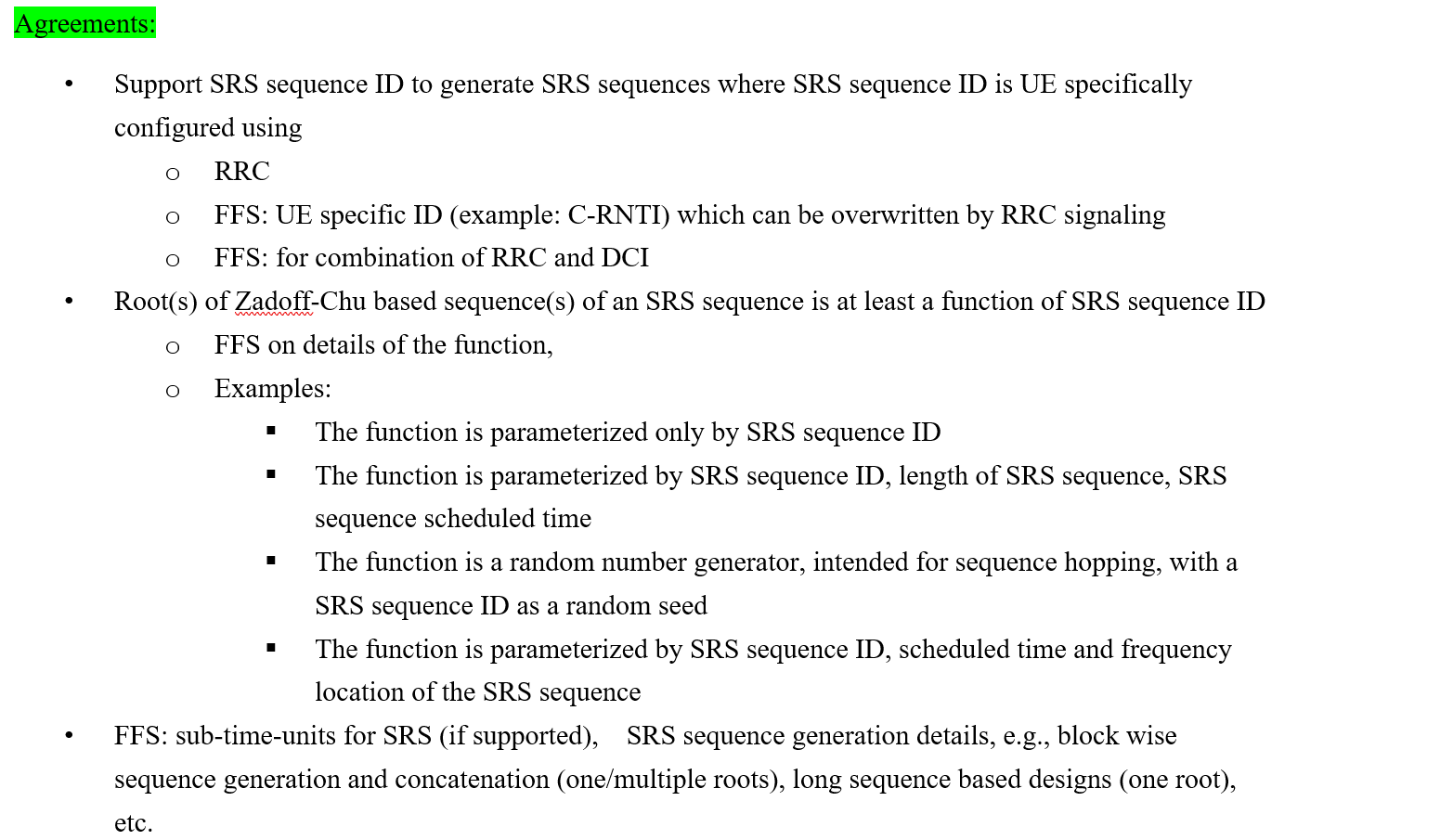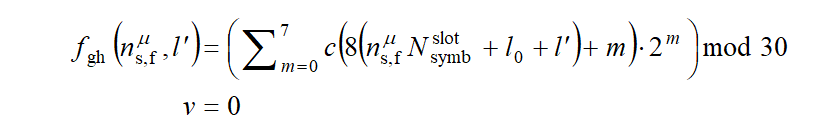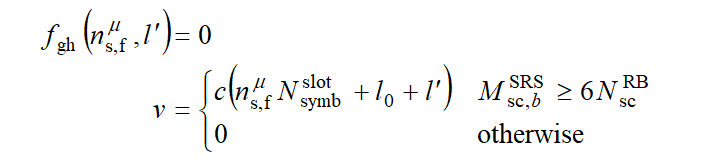# 映射到物理资源

## SRS 频域资源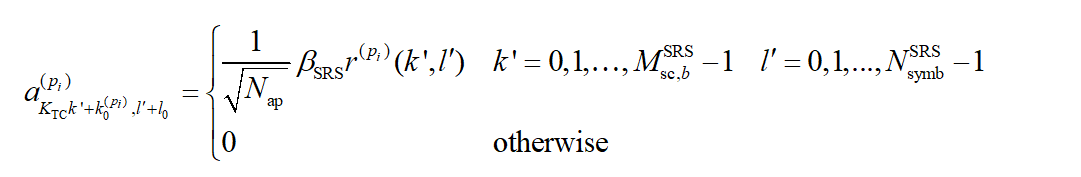SRS 序列长度等于 SRS 资源在一个符号内占用的子载波数目，具体定义为

$M_{\mathrm{sc},b}^{\mathrm{SRS}}=\frac{m_{\mathrm{SRS},b}N_{\mathrm{sc}}^{\mathrm{RB}}}{K_{\mathrm{TC}}}$

$$m_{\mathrm{SRS},b}$$ 通过查 Table 6.4.1.4.3-1 得到，其中 $$b=B_{\mathrm{SRS}}$$$$B_{\mathrm{SRS}}\in\left\{0,1,2,3\right\}$$ 由高层参数 freqHopping 中的字段 b-SRS 给定，$$C_{\mathrm{SRS}}\in\left\{0,1,...,63\right\}$$ 由高层参数 freqHopping 中的 c-SRS 给定。查表时通过 $$C_{\mathrm{SRS}}$$ 选中某一行，再通过 $$B_{\mathrm{SRS}}$$ 选中某一列，即可最终确定 SRS 的带宽信息。 根据 Table 6.4.1.4.3-1，NR 系统支持 64 种 SRS 带宽配置方式，一个 SRS 资源可配置的最小带宽为 4 个 RB，最大带宽为 272 个RB。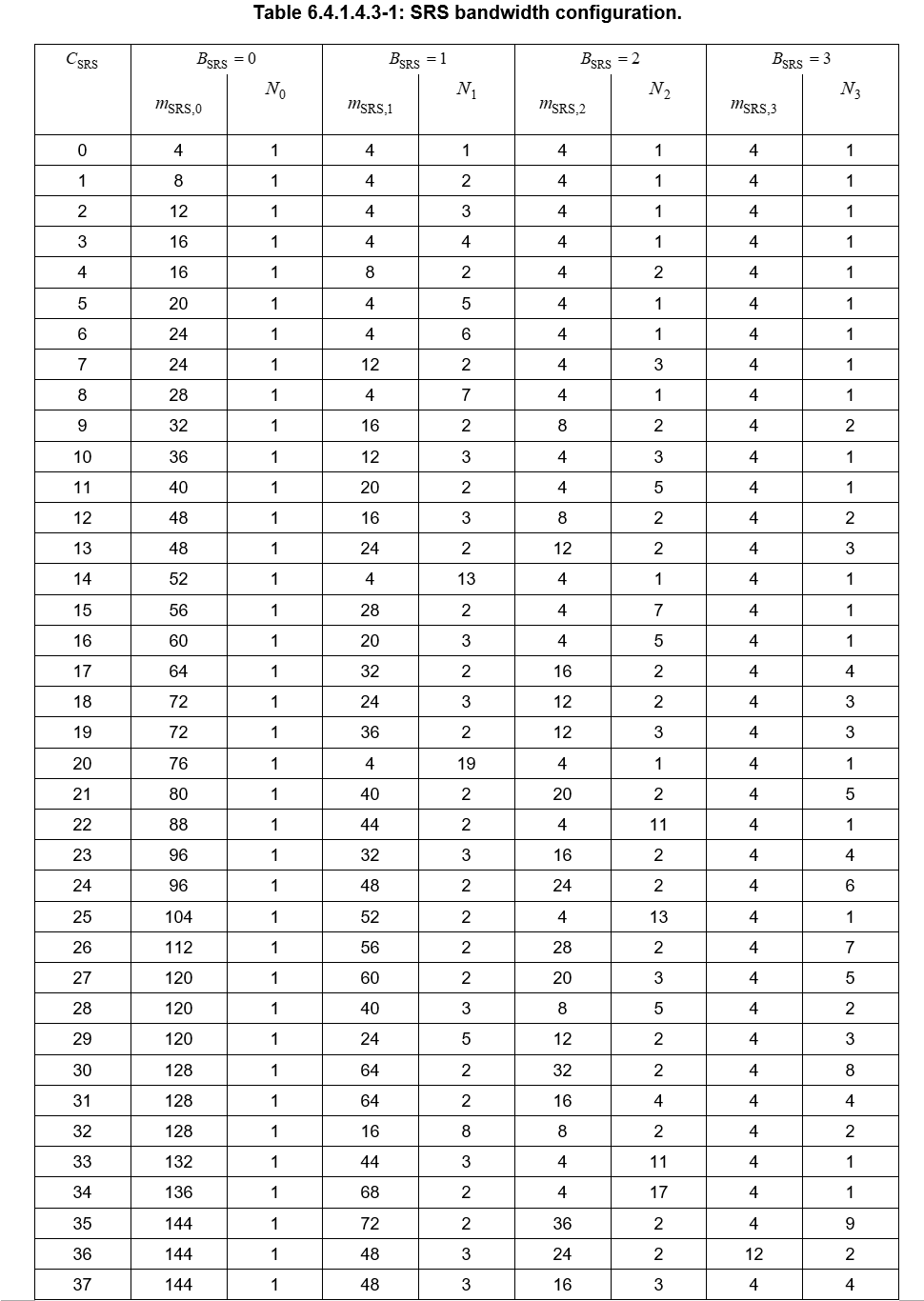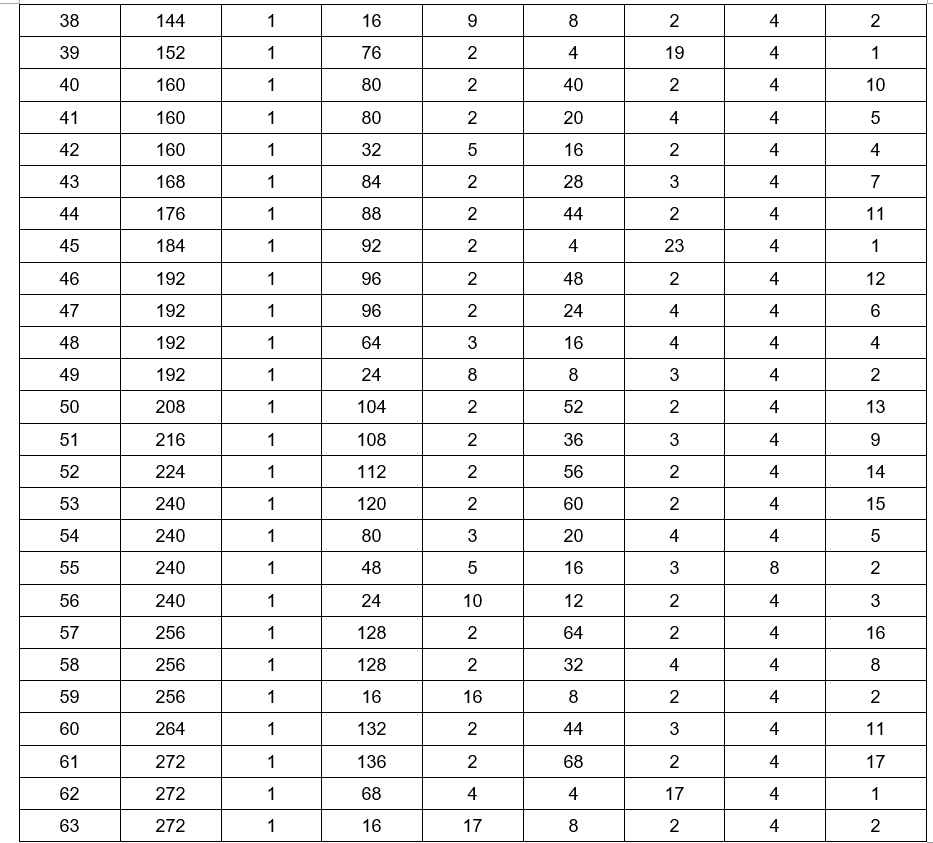$k_0^{(p_i)} = {\bar{k}}_0^{\left(p_i\right)} + \sum_{b=0}^{B_{\mathrm{SRS}}}{K_{\mathrm{TC}}M_{\mathrm{sc},b}^{\mathrm{SRS}}}n_b$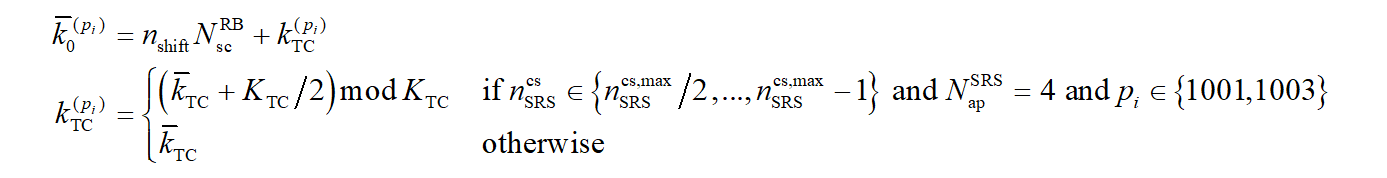SRS 是否跳频由高层参数 freqHopping 中的字段 b-hop 决定。$$b_{\mathrm{hop}}$$ 取值范围 0~3。

$n_b=\left\lfloor\frac{4n_{\mathrm{RRC}}}{m_{\mathrm{SRS,}b}}\right\rfloor\ mod{\ N}_b$

$n_b=\left\{\begin{matrix}\left\lfloor\frac{4n_{\mathrm{RRC}}}{m_{\mathrm{SRS},b}}\right\rfloor\mathrm{\ mod\ }\ N_\mathrm{b}&b\le b_{\mathrm{hop}}\\\left(F_b\left(n_{\mathrm{SRS}}\right)+\left\lfloor\frac{4n_{\mathrm{RRC}}}{m_{\mathrm{SRS},b}}\right\rfloor\right)\mathrm{\ mod\ }\ N_\mathrm{b}&\mathrm{otherwise}\\\end{matrix}\right.$

$F_b(n_{\mathrm{SRS}})=\left\{\begin{matrix}(N_b/2)\left\lfloor\frac{n_{\mathrm{SRS}}mod{\Pi_{b'=b_{\mathrm{hop}}}^b}N_{b'}}{\Pi_{b'=b_{\mathrm{hop}}}^{b-1}N_{b'}}\right\rfloor+\left\lfloor\frac{n_{\mathrm{SRS}}mod{\Pi_{b'=b_{\mathrm{hop}}}^b}N_{b'}}{2\Pi_{b'=b_{\mathrm{hop}}}^{b-1}N_{b'}}\right\rfloor&\mathrm{if\ }N_b\mathrm{\ even\ }\ \\\left\lfloor N_b/2\right\rfloor\left\lfloor n_{\mathrm{SRS}}/\Pi_{b'=b_{\mathrm{hop}}}^{b-1}N_{b'}\right\rfloor&\mathrm{if\ }N_b\mathrm{\ odd}\ \\\end{matrix}\right.$

$$n_{\mathrm{SRS}}$$ 用于 SRS 传输的计数。如果高层参数 resourceType 被配置为 “aperiodic”，则在时隙内发送 $$N_{\mathrm{symb}}^{\mathrm{SRS}}$$ 个符号的 SRS 资源为 $$n_{\mathrm{SRS}}=\left\lfloor\frac{l'}{R}\right\rfloor$$。重复因子 $$R\le\ N_{\mathrm{symb}}^{\mathrm{SRS}}$$ 由高层参数 resourceMapping 中的字段 repetitionFactor 给定。

$n_{\mathrm{SRS}}=\left(\frac{N_{\mathrm{slot}}^{\mathrm{frame},\mu}n_f+n_{\mathrm{s,f}}^\mu-T_{\mathrm{offset}}}{T_{\mathrm{SRS}}}\right)\cdot\left(\frac{N_{\mathrm{symb}}^{\mathrm{SRS}}}{R}\right)+\left\lfloor\frac{l'}{R}\right\rfloor$

# UE 探测过程

UE可以被高层参数 SRS-ResourceSet 配置一个或多个 SRS 资源集。对于每个 SRS 资源集，UE 可以被高层参数 SRS-Resource 配置 $$K\geq1$$ 个 SRS 资源，其中 $$K$$ 的最大值由 UE 能力指示。SRS 资源集的用途由高层参数 SRS-ResourceSet 中的字段 usage 确定。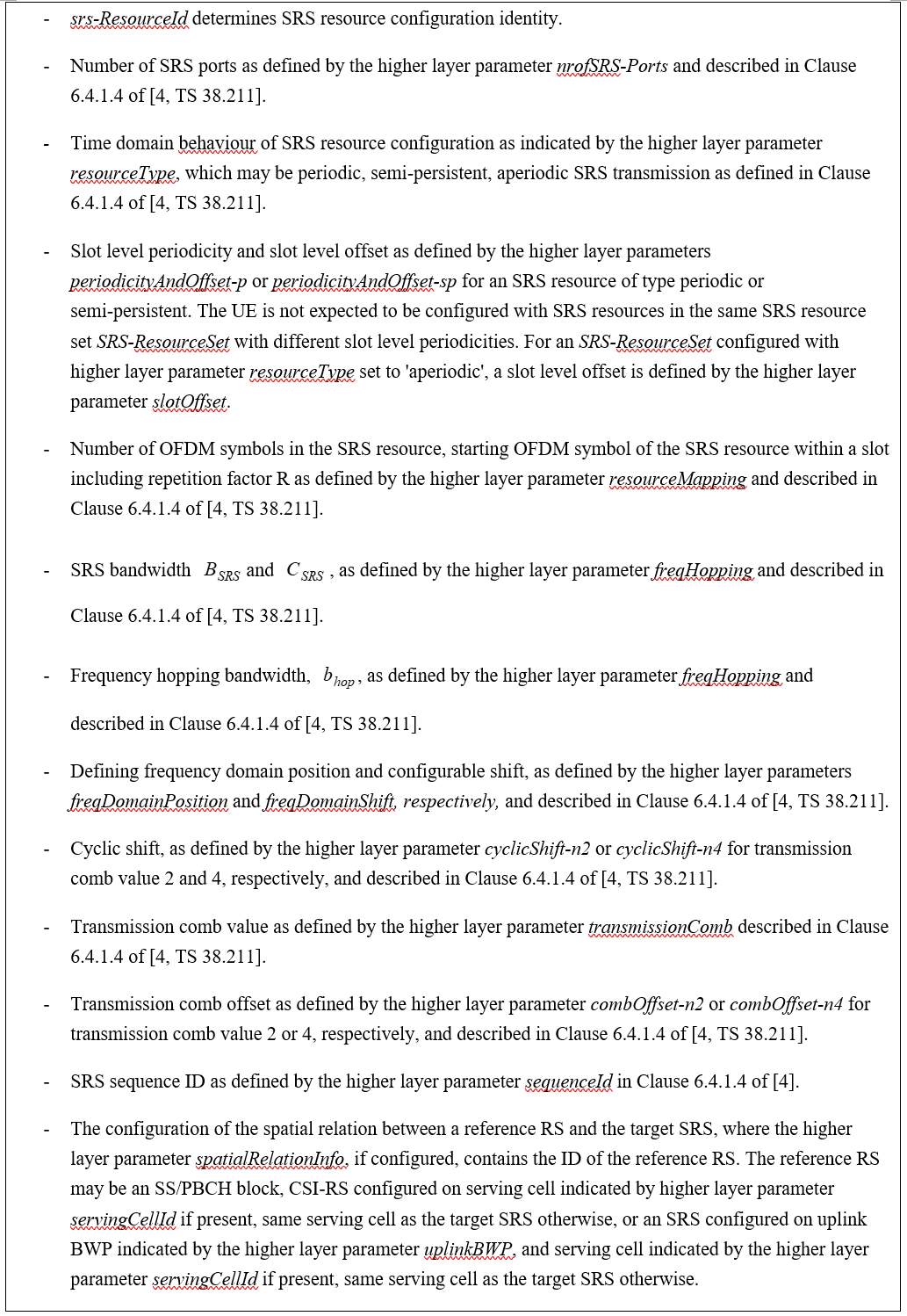UE 由高层参数 SRS-Resource 中的字段 resourceMapping 来配置 SRS 资源，该 SRS 资源位于时隙内最后 6 个符号的区域，并占用 $$N_S\in\left\{1,2,4\right\}$$ 个连续符号。

• 如果配置给 UE 的高层参数spatialRelationInfo 中包含了参考 “ssb-Index”，则 UE 发送目标 SRS 资源时，将使用与接收参考信号 SSB 相同的空间域传输过滤器。

• 如果配置给 UE 的高层参数spatialRelationInfo 中包含了参考 “csi-RS-Index”，则 UE 发送目标 SRS 资源时，将使用与接收周期性 CSI-RS 或者半持续性 CSI-RS 相同的空间域传输过滤器。

• 如果配置给 UE 的高层参数spatialRelationInfo 中包含了参考 “srs”，则 UE 发送目标 SRS 资源时，将使用与接收周期性 SRS 相同的空间域传输过滤器。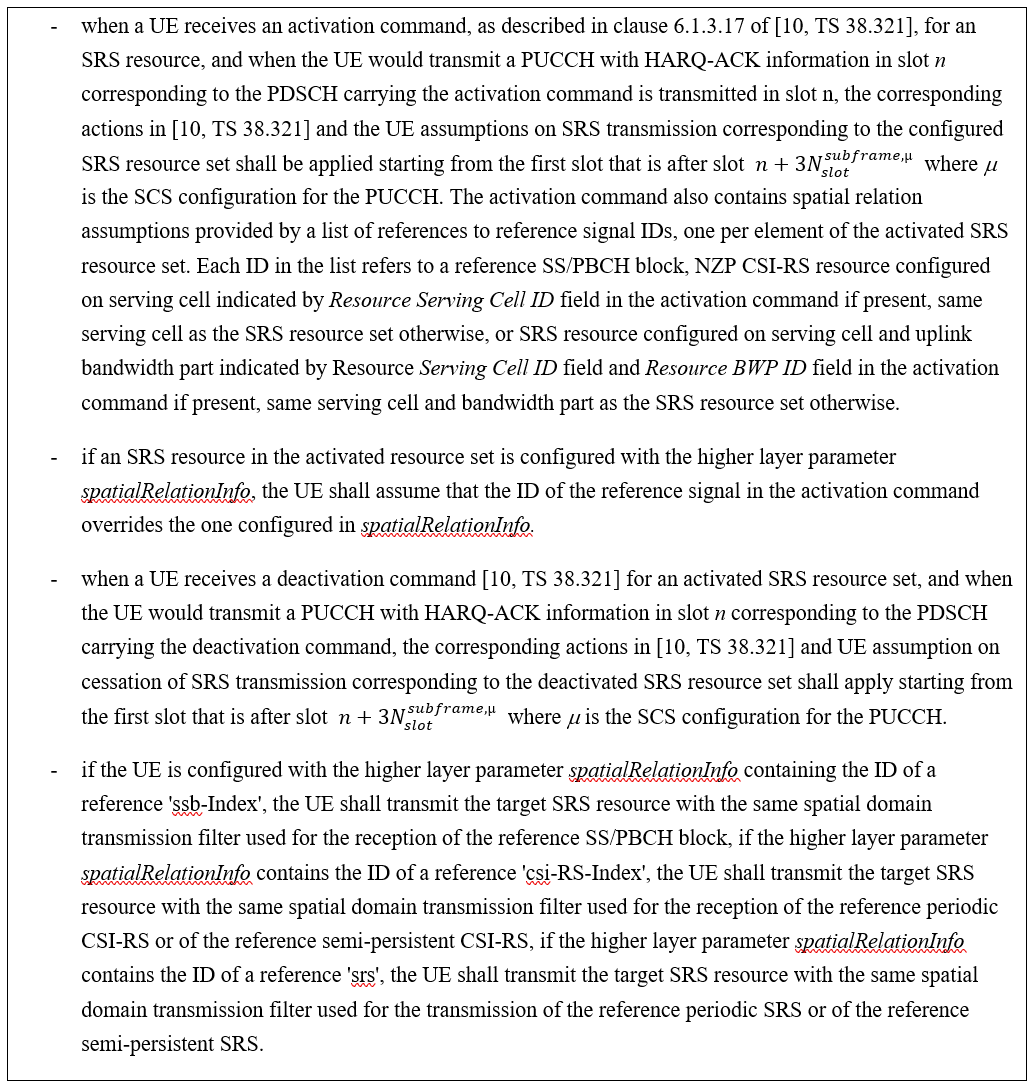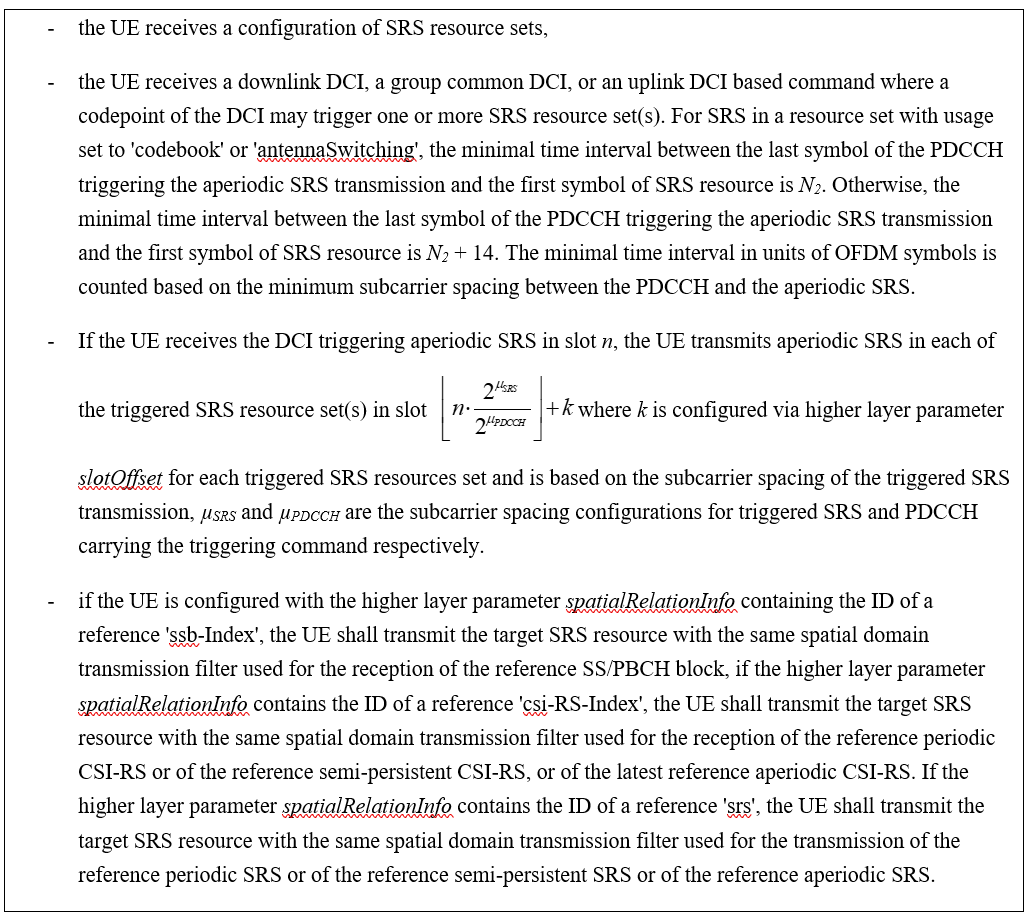DCI format 0_1 和 DCI format 1_1 中的SRS request 字段指示了 Table 7.3.1.1.2-24 中被触发的 SRS 资源集。DCI format 2_3 中 2-bit 的 SRS request 字段，如果 UE 被高层参数 srs-TPC-PDCCH-Group 设置为 “typeB”，则该字段用于指示被触发的 SRS 资源集；如果 UE 被高层参数 srs-TPC-PDCCH-Group 设置为 “typeA”，则该字段用于指示通过高层配置的一组服务小区上进行SRS传输。

# SRS 的冲突处理

PUCCH 和 SRS 在相同载波条件下，

• 当半持续和周期 SRS 与仅携带 CSI 报告的 PUCCH 被配置在相同的符号时，或者当半持续和周期 SRS 与仅携带 L1-RSRP 报告的 PUCCH 被配置在相同的符号时, UE 不应发送 SRS。

• 当半持续性 SRS 或周期性 SRS 或非周期性 SRS 被触发后，与携带 HARQ-ACK 和/或 SR 的 PUCCH 在相同符号发送时，UE 不应发送 SRS。

• 对由于与 PUCCH 重叠而不发送 SRS 的情况，仅丢弃与 PUCCH 重叠的 SRS 符号。

• 非周期性 SRS 被触发后，与携带半持续/周期 CSI 报告或仅携带半持续/周期 L1-RSRP 报告的 PUCCH 在相同符号时，UE 不应发送 PUCCH。

In case of intra-band carrier aggregation or in inter-band CA band combination if simultaneous SRS and PUCCH/PUSCH transmissions are not supported by UE, the UE is not expected to be configured with SRS from a carrier and PUSCH/UL DM-RS/UL PT-RS/PUCCH formats from a different carrier in the same symbol. In case of intra-band carrier aggregation or in inter-band CA band combination if simultaneous SRS and PRACH transmissions are not supported by UE, the UE shall not transmit simultaneously SRS resource(s) from a carrier and PRACH from a different carrier.

## UE SRS 跳频过程

UE可以被配置 Ns = 2 or 4 个相邻符号的非周期 SRS 资源并在 BWP 内进行时隙内跳频，其中当跳频配置为 $$R=1$$ 时，在 $$N_s$$ 个符号上以相等大小的 sub-band 来探测全跳频带宽。UE可以被配置 $$N_s=4$$ 个相邻符号非周期 SRS 资源并在 BWP 内进行时隙内跳频，其中当跳频配置为R = 2时，在两对 R 个相邻的 OFDM 符号之间以相等大小的 sub-band来探测全跳频带宽。SRS 资源的每个天线端口被映射到每对 R 个相邻的 OFDM 符号中相同的子载波集合。

UE可以被配置 $$N_s=1$$ 个符号的周期或半持续 SRS 资源并在 BWP 内进行时隙间跳频，其中 SRS 资源在每个时隙中占据相同的符号位置。UE可以被配置 Ns = 2 or 4 个符号的周期或半持续 SRS 资源并在 BWP 内进行时隙内和时隙间跳频，其中N 个符号的 SRS 资源在每个时隙中占据相同的符号位置。对于 $$N_s=4$$，当跳频配置为 R = 2 时，SRS 资源的每个天线端口通过每个时隙中的资源的两对 R 个相邻的 OFDM 符号映射到不同子载波集合以支持时隙内和时隙间跳频。对于 $$N_s=R$$，当跳频被配置，SRS 资源的每个天线端口映射到每个时隙中的资源的 R 个相邻 OFDM 符号中的相同一组子载波集合以支持时隙间跳频。

## 用于下行 CSI 获取的 UE 探测过程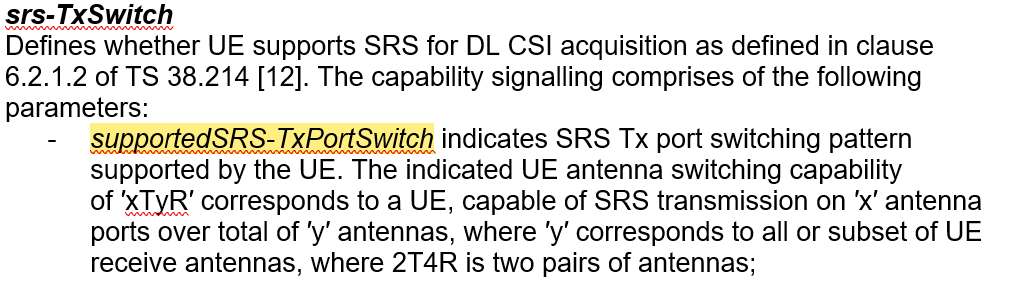• 对于 1T2R，最多配置两个 SRS 资源集。一个 SRS 资源集中包含两个配置在不同 OFDM 符号的 SRS 资源，每个 SRS 资源有 1 个端口。同一个 SRS 资源集中的不同 SRS 资源使用不同的 UE 天线端口（物理天线）分别发送。当配置两个 SRS 资源集时，这两个 SRS 资源集须通过高层参数 resourceType配置为不同的资源类型。

• 对于 2T4R，最多配置带个 SRS 资源集。一个 SRS 资源集中包含两个配置在不同 OFDM 符号的 SRS 资源，每个 SRS 资源有 2 个端口，其中的一个 SRS 资源由两个 UE 天线端口（物理天线）发送，另一个 SRS 资源则从另外两个 UE 天线端口（物理天线）发送。当配置两个 SRS 资源集时，这两个 SRS 资源集须通过高层参数 resourceType配置为不同的资源类型。

• 对于 1T4R，可以配置零个或一个周期或半持续 SRS 资源集。当配置一个周期或半持续 SRS 资源集时，该资源集内包含 4 个 SRS 资源，每个 SRS 资源包含 1 个端口，不同的 SRS 资源配置在不同的 OFDM 符号上，且使用不同的 UE 天线端口（物理天线）发送。

• 对于 1T4R，可以配置零个或两个非周期 SRS 资源集。当配置两个非周期 SRS 资源集时，由于天线切换需要时间，4 个 SRS 资源不能在一个时隙内发完，因此两个非周期 SRS 资源集要在两个时隙内发送。两个 SRS 资源集共包含 4 个 SRS 资源，每个资源集各包含两个 SRS 资源或者一个 SRS 资源集包含 1 个SRS 资源，另外一个 SRS 资源集包含 3 个 SRS 资源，每个 SRS 资源包含一个端口，不同的 SRS 资源通过不同的 UE 物理天线发送。当配置两个非周期 SRS 资源集时，两个 SRS 资源集需配置相同的功率控制参数，包括 SRS-ResourceSet 中的字段 alpha, p0, pathlossReferenceRS 和 srs-PowerControlAdjustmentStates。UE期望高层参数aperiodicSRS-ResourceTrigger的值或每个SRS-ResourceSet中AperiodicSRS-ResourceTriggerList中的条目的值相同，并且每个SRS-ResourceSet中的高层参数slotOffset的值不同 。

• 对于 1T=1R 或 2T=2R 或 4T=4R，最多配置两个 SRS 资源集。每个 SRS 资源集包含 1 个 SRS 资源，每个 SRS 资源的端口数可以为 1，2或4。

Y 的值由 Table 6.2.1.2-1 给出。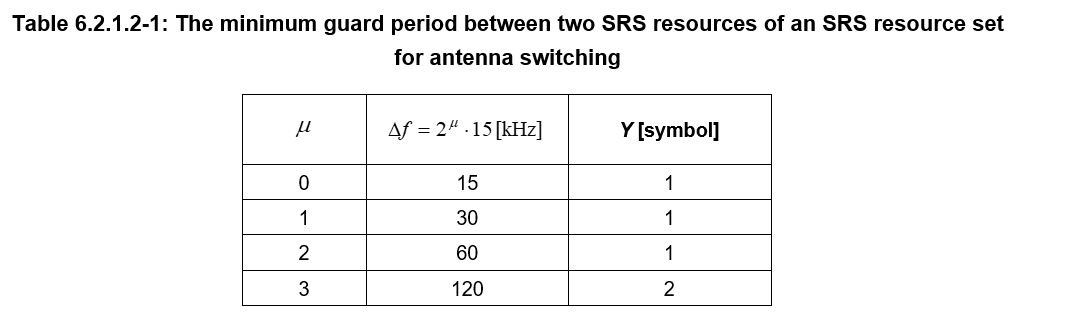# 低峰均比序列

$r_{u,v}^{\left(\alpha,\delta\right)}\left(n\right)=e^{j\alpha n}{\bar{r}}_{u,\nu}\left(n\right), 0≤n<M_{ZC}$

（1）SRS 带宽 4 RB，传输梳齿数目 $$K_{\mathrm{TC}}=2$$, 则 $$\delta={log}_2\left(2\right)=1$$$$\frac{1}{2}\le\frac{4}{2}\le5$$。此时序列长度为 $$M_{\mathrm{ZC}}=\frac{mN_{\mathrm{sc}}^{\mathrm{RB}}}{2^\delta}=4\ast12/2=24$$

（2）SRS 带宽 4 RB，传输梳齿数目 $$K_{\mathrm{TC}}=4$$, 则 $$\delta={log}_2\left(4\right)=2$$$$\frac{1}{2}\le\frac{4}{4}\le5$$。此时序列长度为 $$M_{\mathrm{ZC}}=\frac{mN_{\mathrm{sc}}^{\mathrm{RB}}}{2^\delta}=4\ast12/4=12$$

（3）SRS 带宽 8 RB，传输梳齿数目 $$K_{\mathrm{TC}}=4$$, 则 $$\delta={log}_2\left(4\right)=2$$$$\frac{1}{2}\le\frac{8}{4}\le5$$。此时序列长度为 $$M_{\mathrm{ZC}}=\frac{mN_{\mathrm{sc}}^{\mathrm{RB}}}{2^\delta}=8\ast12/4=24$$

## 长度大于等于 36 的基序列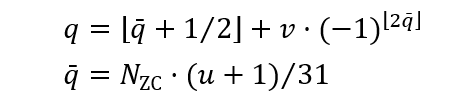ZC 序列长度 $$N_{\mathrm{ZC}}$$ 是满足 $$N_{\mathrm{ZC}}<M_{\mathrm{ZC}}$$ 的最大质数。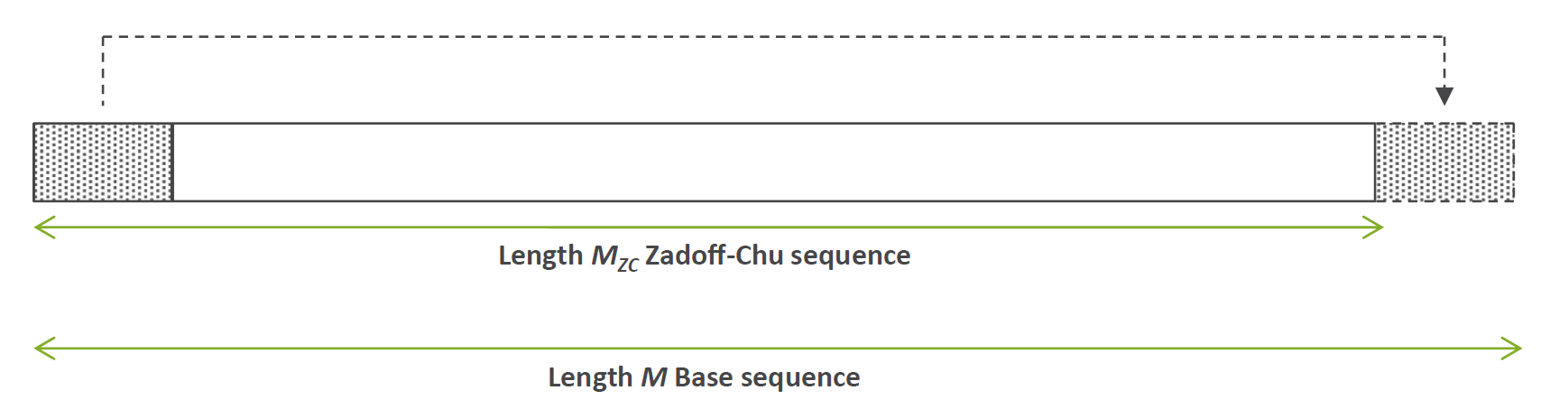ZC 序列的长度通常小于基序列的长度，剩余的那部分基序列由 ZC 序列循环扩展得到，首尾相接。例如基序列长度 $$M_{\mathrm{ZC}}=36$$，则 ZC 序列长度为 $$N_{\mathrm{ZC}}=31$$。通过对基序列进行循环移位可得到更多的 ZC 序列，当 $$N_{\mathrm{ZC}}=31$$ 时，可用的 ZC 序列有 30 个。可用的 ZC 序列数目为 $$N_{\mathrm{ZC}}-1$$

## 长度小于 36 的基序列

${\bar{r}}_{u,\nu}\left(n\right)=\begin{matrix}e^\frac{j\phi\left(n\right)\pi}{4},&0\le n\le M_{\mathrm{ZC}}-1\\\end{matrix}$

${\bar{r}}_{u,\nu}\left(n\right)=\begin{matrix}e^{-j\frac{\pi(u+1)(n+1)(n+2)}{31}},&0\le n\le M_{\mathrm{ZC}}-1\\\end{matrix}$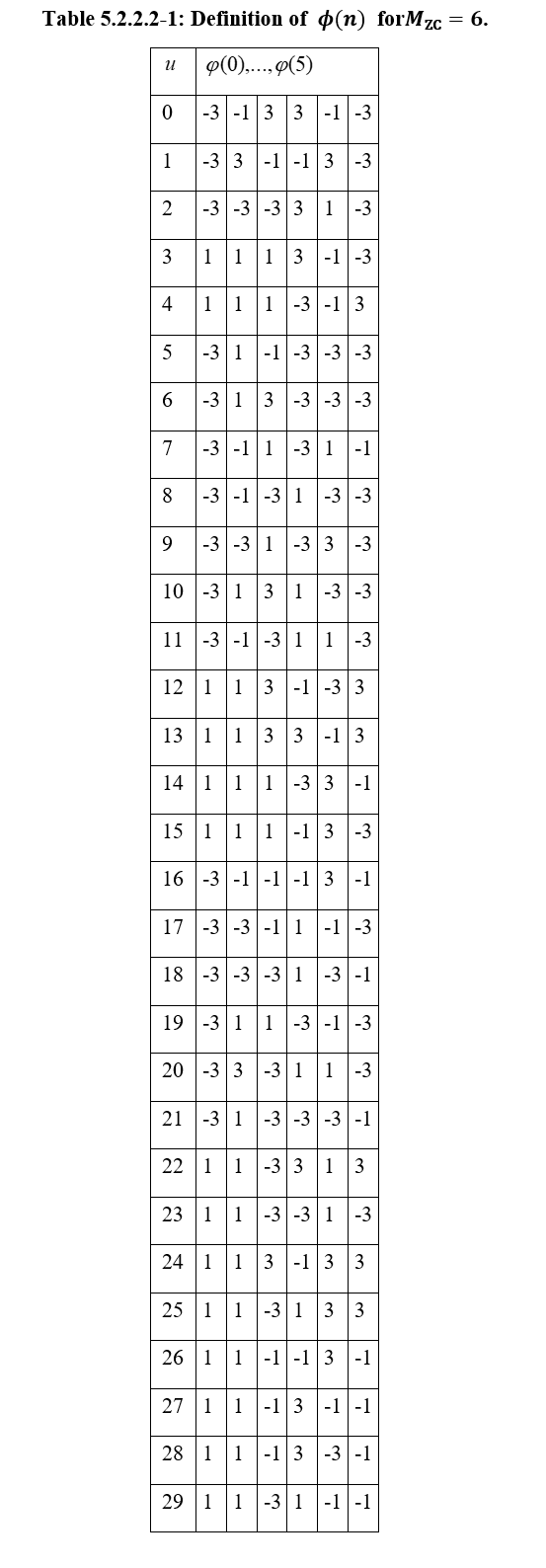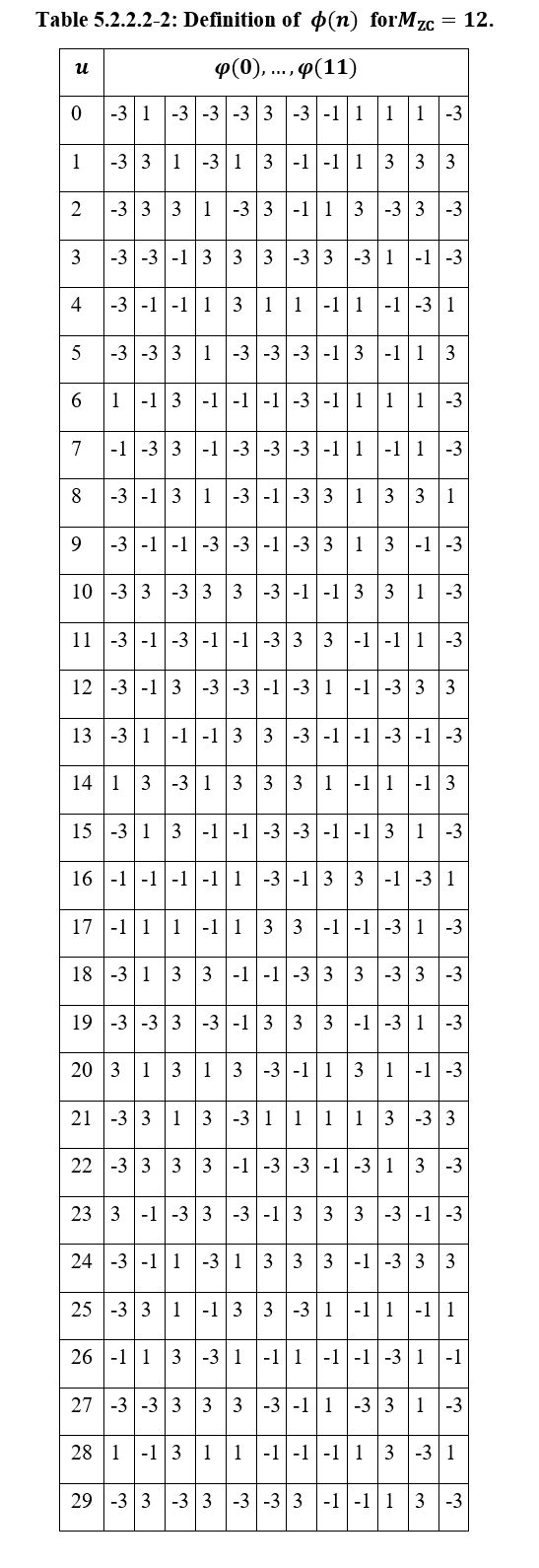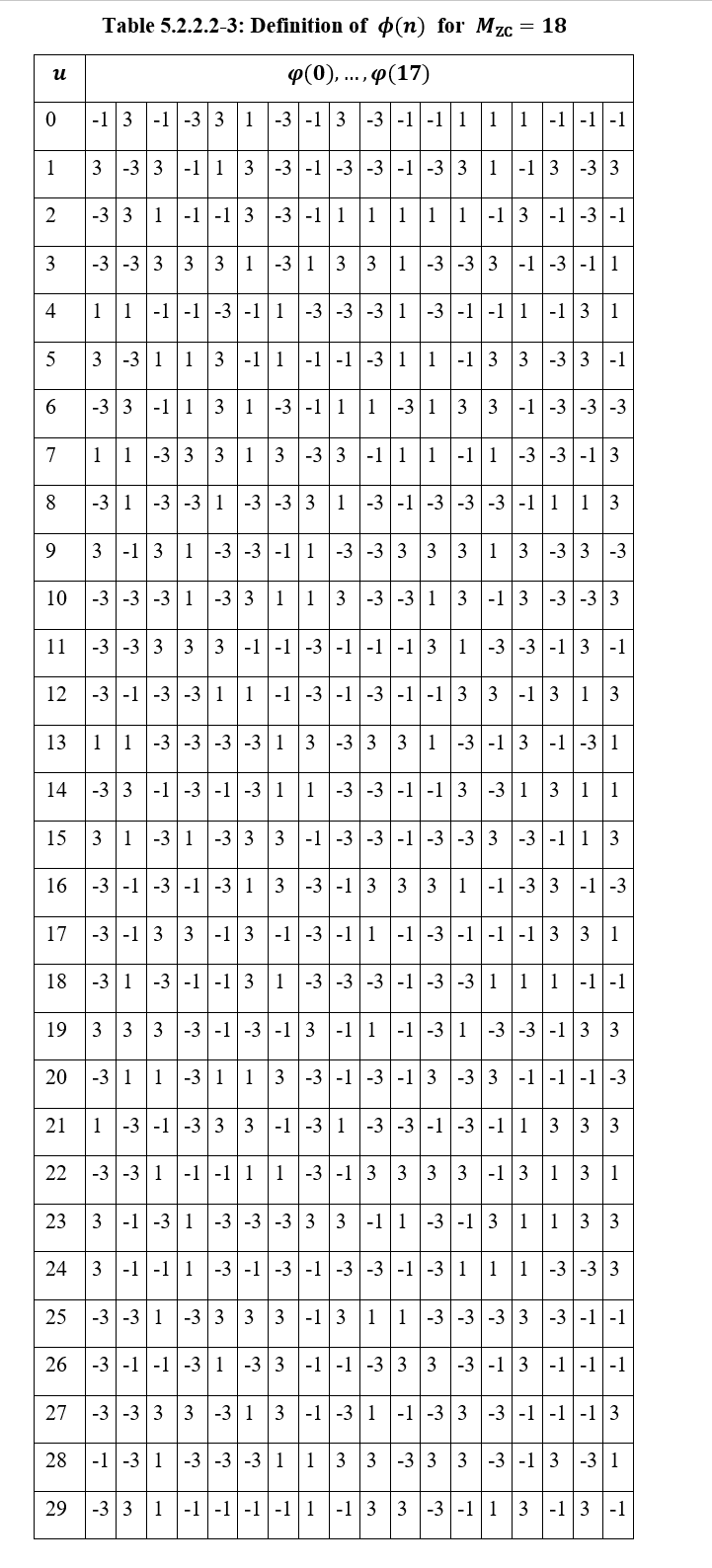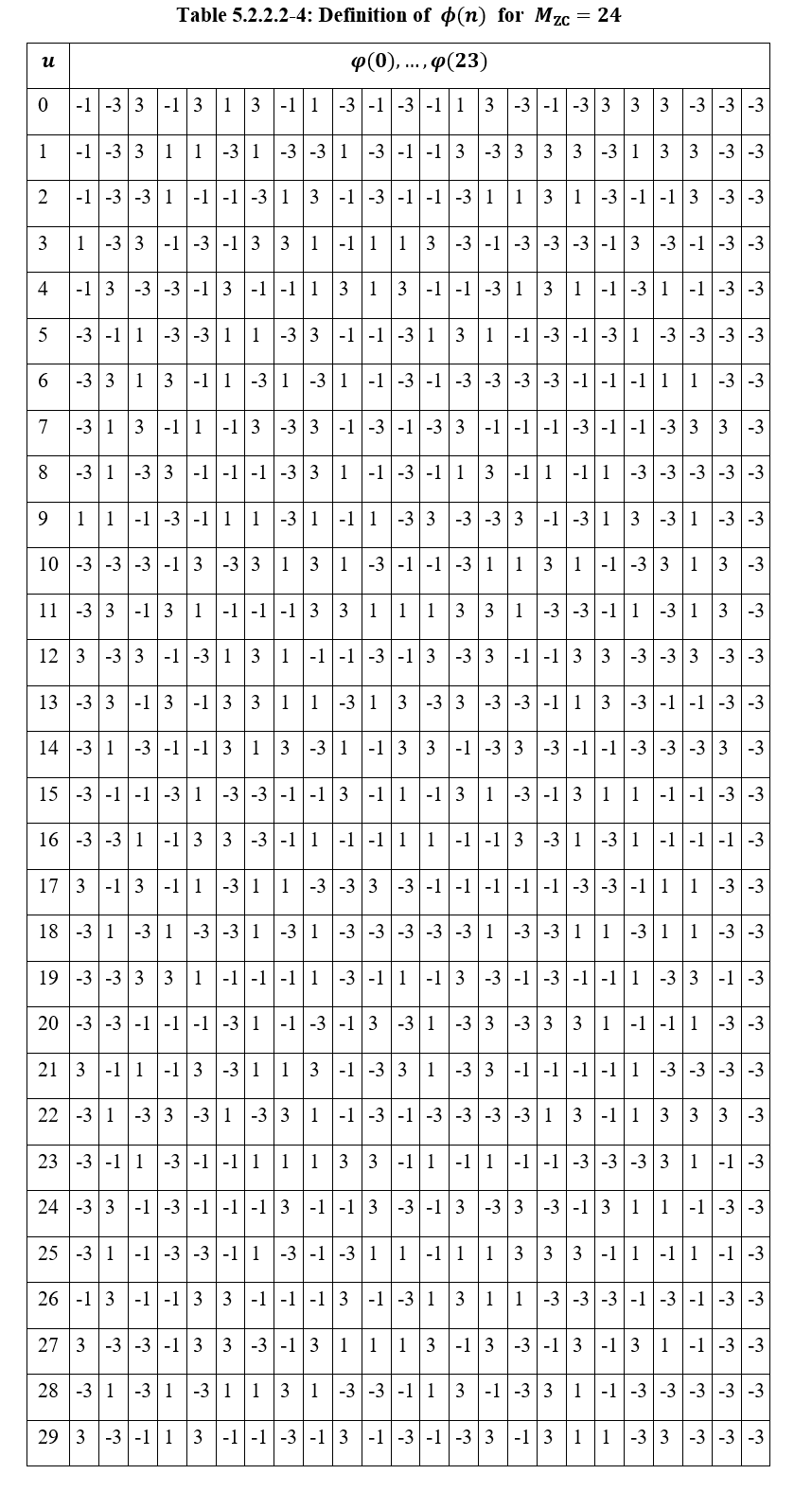# 为什么是 ZC 序列

1962 年 Zadoff 和 Frank 提出了一种循环自相关为零的多相编码（polyphase codes），但编码的长度受限于 p^2。十年后（1972），Chu 基于 Zadoff 和 Frank 的研究成果，提出了一种新的序列构造方法，依然具有完美的零相关性，但序列长度没有限制。这种新的序列就是 Zadoff-Chu 序列。又数十年后，它应用在4G 和 5G 的参考信号中。

Zadoff-Chu 序列一个关键特点是经过离散傅里叶变换，生成的新序列依然是 Zadoff-Chu 序列。Zadoff-Chu 序列的另一个特点是序列在时域和频域的幅度都是恒定的。时域信号的幅度恒定有助于提高功放效率。频域信号的幅度恒定意味着序列经过任意非零循环移位与原序列零相关。这就是说，同一个 Zadoff-Chu 序列在时域上经过不同的循环移位所产生的两个序列信号之间是正交的。时域的循环移位等效于在频域上进行连续的相位旋转。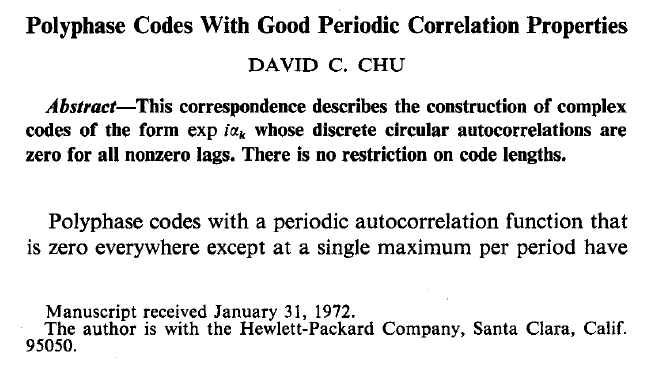David C. Chu 在 1972 年从数学上证明了 Zadoff-Chu 序列具有完美的循环自相关特性。

ZC 序列根据序列长度 N 为偶或奇定义如下：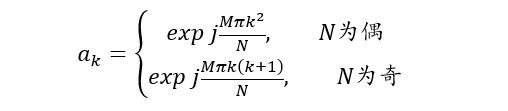$x_0=\sum_{k=0}^{N-1}{a_ka_k^\ast}$

$x_m=\sum_{k=0}^{N-m-1}{a_ka_{k+m}^\ast}+\sum_{k=N-m}^{N-1}{a_ka_{k+m-N}^\ast},\ \ j=1,2,...,N-1$

Output: